Objective :
To find, in a given database, the form closest to the binary image that will be given as input by the user.
Primary language: Language C.

Principle :
The learning phase will consist in calculating the centered moments of each reference form of a database, which needs to be constituted according to the chosen application. In the recognition phase, we compare the moments of the image given by the user with those of the database and deduce the most likely form. Moreover, the centered moments are not invariant in rotation, translation and scaling. To solve this problem, we use the moments of Legendre.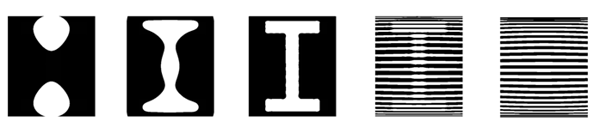Methods of Resolution :
Geometric moments of an image :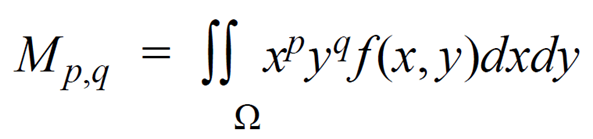Centered and standardized moments :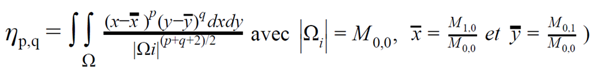Legendre polynomial of order n :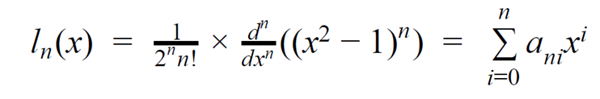Moments of Legendre :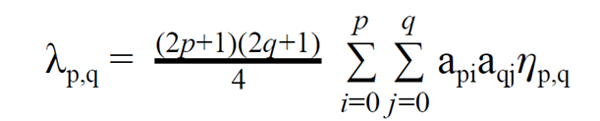This allows us to rebuild the image through a simple method.
The "reconstructed" value of the pixel (x, y) :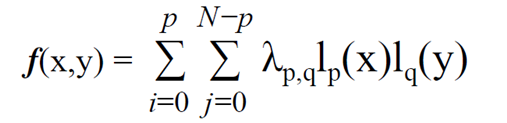To determine whether an image corresponds to an image of the database, we calculate the Euclidean difference between their Legendre moment matrices.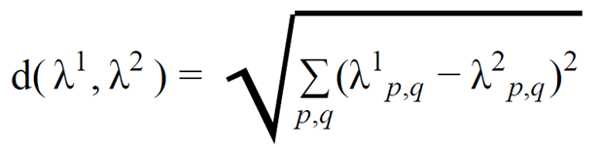We take the image in the database that gives the smallest Euclidean difference as the image that corresponds to the given image.

C Language Implementation :

Results :
By manually testing, the maximum orders for each image were determined :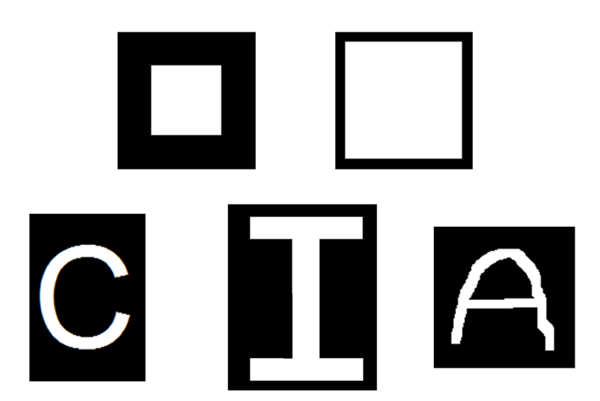Square = 50

"I" = 37
"A" = 37
"C" = 37

With our final program we have seen that we can decrease the normalized mean square error of our image, in other words, we can improve the resolution of our reconstructed image by increasing the N reconstruction order. On the other hand, starting from a certain order of reconstruction, the standardized mean square error increases very rapidly.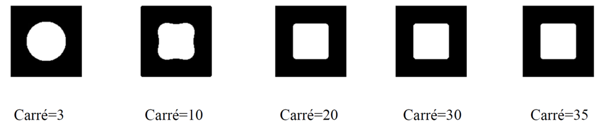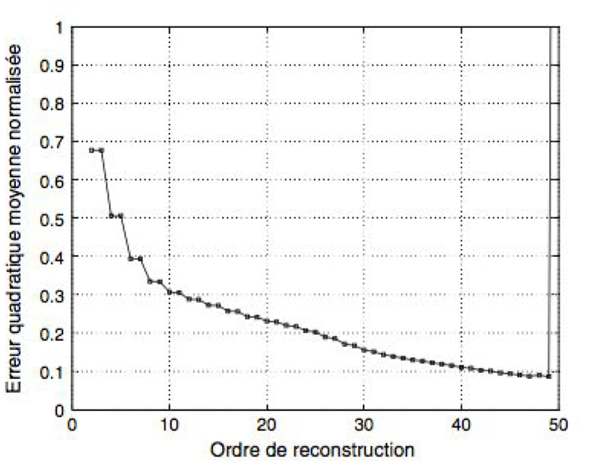Possible Applications :